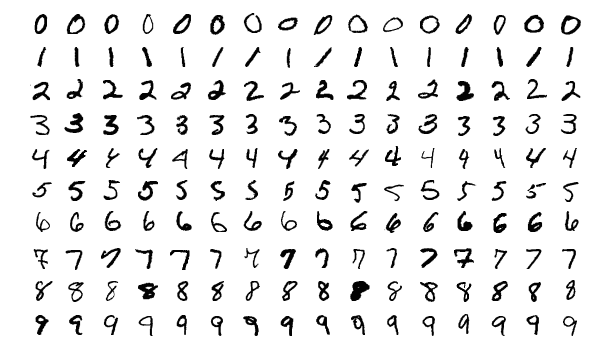# PYTORCH DATA LOADERS — 4 TypesIn this article I will show you how to setup Data loaders and Transformers in Pytorch, You need to import below for the same exercise.

In this article I will show you how to setup Data loaders and Transformers in Pytorch, You need to import below for the same exercise

import torchvision

import torch

import os

import matplotlib.pyplot as plt

import numpy as np

## 1. Define the Transform

Image Resize (256,256) or Any other size

Convert to Pytorch Tensors

Normalize the Image by calling torchvision.transform.Normalize

transform_img = torchvision.transforms.Compose([torchvision.transforms.Resize((256, 256)),

torchvision.transforms.ToTensor(),

torchvision.transforms.Normalize(mean=[0.485],std=[0.229])])

## 2. Create the DataSet from torchvision.datasets

Set some Directory Path, download = True will download the data into the directory specified, transform should be set to transform defined above

dir_path= ‘C:\Users\Asus\pytorch-basics-part2’

dataset_mnist_train = torchvision.datasets.MNIST(dir_path, train=True, transform=transform_img,

You can index this Dataset, dataset_mnist_train[i] will contain the Tuple of (Image, Label).

## PyTorch For Deep Learning

Pytorch is a Deep Learning Library Devoloped by Facebook. it can be used for various purposes such as Natural Language Processing , Computer Vision, etc

## PyTorch For Deep Learning — Confusion Matrix

This is a regular classification problem with PyTorch and this is exactly like the one in the previous post of the “PyTorch for Deep Learning” series. The Reason for doing writing the post is for some more reference to classification problem and better understanding.

## PyTorch for Deep Learning

PyTorch is a library in Python which provides tools to build deep learning models. What python does for programming PyTorch does for deep learning.

## Learn Transfer Learning for Deep Learning by implementing the project.

Project walk-through on Convolution neural networks using transfer learning. From 2 years of my master’s degree, I found that the best way to learn concepts is by doing the projects.

## PyTorch for Deep Learning | Data Science | Machine Learning | Python

PyTorch for Deep Learning | Data Science | Machine Learning | Python. PyTorch is a library in Python which provides tools to build deep learning models. What python does for programming PyTorch does for deep learning. Python is a very flexible language for programming and just like python, the PyTorch library provides flexible tools for deep learning.